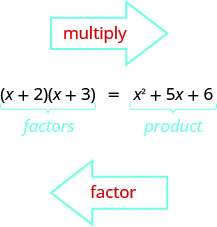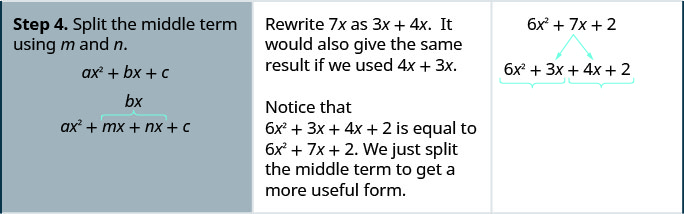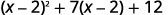# 6.3: 因子三项式

•• OpenStax
• OpenStax
$$\newcommand{\vecs}{\overset { \rightharpoonup} {\mathbf{#1}} }$$ $$\newcommand{\vecd}{\overset{-\!-\!\rightharpoonup}{\vphantom{a}\smash {#1}}}$$$$\newcommand{\id}{\mathrm{id}}$$ $$\newcommand{\Span}{\mathrm{span}}$$ $$\newcommand{\kernel}{\mathrm{null}\,}$$ $$\newcommand{\range}{\mathrm{range}\,}$$ $$\newcommand{\RealPart}{\mathrm{Re}}$$ $$\newcommand{\ImaginaryPart}{\mathrm{Im}}$$ $$\newcommand{\Argument}{\mathrm{Arg}}$$ $$\newcommand{\norm}{\| #1 \|}$$ $$\newcommand{\inner}{\langle #1, #2 \rangle}$$ $$\newcommand{\Span}{\mathrm{span}}$$ $$\newcommand{\id}{\mathrm{id}}$$ $$\newcommand{\Span}{\mathrm{span}}$$ $$\newcommand{\kernel}{\mathrm{null}\,}$$ $$\newcommand{\range}{\mathrm{range}\,}$$ $$\newcommand{\RealPart}{\mathrm{Re}}$$ $$\newcommand{\ImaginaryPart}{\mathrm{Im}}$$ $$\newcommand{\Argument}{\mathrm{Arg}}$$ $$\newcommand{\norm}{\| #1 \|}$$ $$\newcommand{\inner}{\langle #1, #2 \rangle}$$ $$\newcommand{\Span}{\mathrm{span}}$$$$\newcommand{\AA}{\unicode[.8,0]{x212B}}$$

##### 学习目标

• 形式的因子三项式$$x^2+bx+c$$
• $$ax^2+bx+c$$使用反复试验对形式的三项式进行分数
• $$ax^2+bx+c$$使用 '$$ac$$' 方法对形式的三项式进行因子分数
• 使用替换的因子

1. 找出 72 的所有因子。
如果你错过了这个问题，请查看 [链接]
2. 查找产品:$$(3y+4)(2y+5)$$.
如果你错过了这个问题，请查看 [链接]
3. 简化：$$−9(6);\space −9(−6)$$
如果你错过了这个问题，请查看 [链接]

## 形式的因子三项式$$x^2+bx+c$$$$(x+m)(x+n)$$ 用铝箔找到产品。 $$x^{2}+m x+n x+m n$$ 将GCF从中间值中考虑在内。 $$x^{2}+(m+n) x+m n$$ 我们的三项式是这样的$$x^2+bx+c$$。 $$\overbrace{x^{2}+(m+n) x+m n}^{\color{red}x^{2}+b x+c}$$

##### 示例$$\PageIndex{1}$$: How to Factor a Trinomial of the form $$x^2+bx+c$$##### 示例$$\PageIndex{2}$$

$$(q+4)(q+6)$$

##### 示例$$\PageIndex{3}$$

$$(t+2)(t+12)$$

1. 将因子写成两个二项式，第一个项为 x$$\quad \begin{array} {l} x^2+bx+c \\ (x\quad)(x\quad) \end{array}$$
2. 找两个数字$$m$$然后$$n$$那个
• 乘以$$c$$$$m·n=c$$
• 添加到$$b$$$$m+n=b$$
3. 使用$$m$$$$n$$作为因子的最后一个术语。 $$\quad (x+m)(x+n)$$
4. 通过乘以因子进行检查。

##### 示例$$\PageIndex{4}$$

$$\begin{array} {ll} &y^2−11y+28 \\ \text{Write the factors as two binomials with first terms }y. &( y \quad )( y \quad ) \\ \text{Find two numbers that: multiply to }28\text{ and add to }−11.\end{array}$$

\ (28\)” data-valign= “top” >$$−1,\space −28$$

$$−2,\space −14$$

$$−4,\space −7$$
$$−1+(−28)=−29$$

$$−2+(−14)=−16$$

$$−4+(−7)=−11^∗$$

$$\begin{array} {ll} \text{Use }−4,\space −7\text{ as the last terms of the binomials.} &(y−4)(y−7) \\ \text{Check:} & \\ \hspace{30mm} (y−4)(y−7) & \\ \hspace{25mm} y^2−7y−4y+28 & \\ \hspace{30mm} y^2−11y+28\checkmark & \end{array}$$

##### 示例$$\PageIndex{5}$$

$$(u−3)(u−6)$$

##### 示例$$\PageIndex{6}$$

$$(y−7)(y−9)$$

##### 示例$$\PageIndex{7}$$

$$\begin{array} {ll} &2x+x^2−48 \\ \text{First we put the terms in decreasing degree order.} &x^2+2x−48 \\ \text{Factors will be two binomials with first terms }x. &(x\quad)(x\quad) \end{array}$$

−48−48 的因子 因子总和
$$−1,\space 48$$
$$−2,\space 24$$
$$−3,\space 16$$
$$−4,\space 12$$
$$−6,\space 8$$
$$−1+48=47$$
$$−2+24=22$$
$$−3+16=13$$
$$−4+12=8$$
$$−6+8=2^∗$$

$$\begin{array} {ll} \text{Use }−6,\space 8\text{ as the last terms of the binomials.} &(x−6)(x+8) \\ \text{Check:} & \\ \hspace{30mm} (x−6)(x+8) & \\ \hspace{25mm} x^2−6q+8q−48 & \\ \hspace{30mm} x^2+2x−48\checkmark & \end{array}$$

##### 示例$$\PageIndex{8}$$

$$(m+3)(m+6)$$

##### 示例$$\PageIndex{9}$$

$$(n−3)(n−4)$$

##### 示例$$\PageIndex{10}$$

$$\begin{array} {ll} &r^2−8rs−9s^2 \\ \text{Note that the first terms are }r,\text{last terms contain }s. &(r\quad s)(r\quad s) \\ \text{Find the numbers that multiply to }−9\text{ and add to }−8. \end{array}$$

\ (−9\)” data-valign= “top” >$$1,\space −9$$ $$−1+9=8$$
\ (−9\)” data-valign= “top” >$$−1,\space 9$$ $$1+(−9)=−8^∗$$
\ (−9\)” data-valign= “top” >$$3,\space −3$$ $$3+(−3)=0$$

$$\begin{array} {ll} \text{Use }1,\space -9\text{ as coefficients of the last terms.} &(r+s)(r−9s) \\ \text{Check:} & \\ \hspace{30mm} (r−9s)(r+s) & \\ \hspace{25mm} r^2+rs−9rs−9s^2 & \\ \hspace{30mm} r^2−8rs−9s^2\checkmark & \end{array}$$

##### 示例$$\PageIndex{11}$$

$$(a−b)(a−10b)$$

##### 示例$$\PageIndex{12}$$

$$(m−n)(m−12n)$$

##### 示例$$\PageIndex{13}$$

$$\begin{array} {ll} &u^2−9uv−12v^2 \\ \text{Note that the first terms are }u,\text{ last terms contain }v. &(u\quad v)(u\quad v) \\ \text{Find the numbers that multiply to }−12\text{ and add to }−9. & \end{array}$$

\ (−12\)” data-valign= “top” >$$1,−12$$
$$−1,12$$
$$2,−6$$
$$−2,6$$
$$3,−4$$
$$−3,4$$
$$1+(−12)=−11$$
$$−1+12=11$$
$$2+(−6)=−4$$
$$−2+6=4$$
$$3+(−4)=−1$$
$$−3+4=1$$

##### 分解形式为三项式的策略$$x^2+bx+c$$

 $$x^{2}+b x+c$$ $$(x+m)(x+n)$$ 何时$$c$$为正数$$n$$，$$m$$且符号相同。 $$b$$阳性 $$b$$负面的 $$m,n$$阳性 $$m,n$$负面的 $$x^{2}+5 x+6$$ $$x^{2}-6 x+8$$ $$(x+2)(x+3)$$ $$(x-4)(x-2)$$ 同样的迹象 同样的迹象 何时$$c$$为负数，$$m$$且$$n$$具有相反的符号。 $$x^{2}+x-12$$ $$x^{2}-2 x-15$$ $$(x+4)(x-3)$$ $$(x-5)(x+3)$$ 相反的迹象 相反的迹象

## 使用反复试验的因子三项式 ax2 + bx + c

##### 示例$$\PageIndex{16}$$

$$\begin{array} {lll} \text{Is there a greatest common factor?} &\qquad &4x^3+16x^2−20x \\ \quad \text{Yes, }GCF=4x.\text{ Factor it.} & &4x(x^2+4x−5) \\ & & \\ & & \\ \text{Binomial, trinomial, or more than three terms?} & & \\ \quad \text{It is a trinomial. So “undo FOIL.”} & &4x(x\quad)(x\quad) \\ & & \\ & & \\ \text{Use a table like the one shown to find two numbers that} & &4x(x−1)(x+5) \\ \text{multiply to }−5\text{ and add to }4. & & \\ & & \\ & & \end{array}$$

\ (−5\)” data-valign= “top” >$$−1,5$$
$$1,−5$$
$$−1+5=4^∗$$
$$1+(−5)=−4$$

$$\begin{array} {l} \text{Check:}\\ \hspace{27mm}4x(x−1)(x+5) \\ \hspace{25mm} 4x(x^2+5x−x−5) \\ \hspace{30mm} 4x(x^2+4x−5) \\ \hspace{25mm} 4x^3+16x2−20x\checkmark \end{array}$$

##### 示例$$\PageIndex{17}$$

$$5x(x−1)(x+4)$$

##### 示例$$\PageIndex{18}$$

$$6y(y−2)(y+5)$$

$3x^2+5x+2\nonumber$$(\quad)(\quad)\nonumber$$(x+1)(3x+2)\nonumber$$3x^2+2x+3x+2\nonumber$$3x^2+5x+2\checkmark\nonumber$

$3x^2+5x+2\nonumber$$(x+1)(3x+2)\nonumber$

##### 示例$$\PageIndex{19}$$: How to Factor a Trinomial Using Trial and Error##### 示例$$\PageIndex{20}$$

$$(a+1)(2a+3)$$

##### 示例$$\PageIndex{21}$$

$$(b+1)(4b+1)$$

##### 形式的@@ 因子三项式$$ax^2+bx+c$$ USING TRIAL AND ERROR.
1. 根据需要按度数的降序写出三项式。
2. 将任何 GCF 考虑在内。
3. 找出第一个项的所有因子对。
4. 找出第三项的所有因子对。
5. 测试所有可能的因子组合，直到找到正确的乘积。
6. 乘法检查。

##### 示例$$\PageIndex{22}$$

 三项式已经按降序排列了。找出第一个术语的因素。找出最后一个学期的因素。 考虑一下这些迹象。 由于最后一个学期为正$$5$$，其因子必须均为 正数或两者均为负数。 中间项的系数为负，因此我们使用负因子。$$6b^2−13b+5$$

\ (6b^2−13b+5\) 可能的因素” data-valign= “top” >$$(b−1)(6b−5)$$ \ (6b^2−13b+5\) 产品” data-valign= “top” >$$6b^2−11b+5$$
\ (6b^2−13b+5\) 可能的因素” data-valign= “top” >$$(b−5)(6b−1)$$ \ (6b^2−13b+5\) 产品” data-valign= “top” >$$6b^2−31b+5$$
\ (6b^2−13b+5\) 可能的因素” data-valign= “top” >$$(2b−1)(3b−5)$$ \ (6b^2−13b+5\) 产品” data-valign= “middle” >$$6b^2−13b+5^∗$$
\ (6b^2−13b+5\) 可能的因素” data-valign= “top” >$$(2b−5)(3b−1)$$ \ (6b^2−13b+5\) 产品” data-valign= “middle” >$$6b^2−17b+5$$

$$\begin{array} {ll} \text{The correct factors are those whose product} & \\ \text{is the original trinomial.} &(2b−1)(3b−5) \\ \text{Check by multiplying:} & \\ \hspace{50mm} (2b−1)(3b−5) & \\ \hspace{47mm} 6b^2−10b−3b+5 & \\ \hspace{50mm} 6b^2−13b+5\checkmark & \end{array}$$

##### 示例$$\PageIndex{23}$$

$$(2x−3)(4x−1)$$

##### 示例$$\PageIndex{24}$$

$$(2y−7)(5y−1)$$

##### 示例$$\PageIndex{25}$$

 三项式已经按降序排列了。找出第一个术语的因素。找出最后一个学期的因素。 考虑一下这些迹象。 由于 15 为正而中间 项系数为负，因此我们使用负因子。$$\begin{array} {ll} \text{The correct factors are those whose product is} & \\ \text{the original trinomial.} &(2x−3y)(9x−5y) \\ \text{Check by multiplying:} & \\ & \\ & \\ & \\ \hspace{50mm} (2x−3y)(9x−5y) & \\ \hspace{45mm}18x^2−10xy−27xy+15y^2 & \\ \hspace{47mm}18x^2−37xy+15y^2\checkmark & \end{array}$$

##### 示例$$\PageIndex{26}$$

$$(3x+2y)(6x−5y)$$

##### 示例$$\PageIndex{27}$$

$$(3x+y)(10x−21y)$$

##### 示例$$\PageIndex{28}$$注意最大的共同因素，所以请先将其考虑在内。将三项式分解为因子。$$\begin{array} {ll} \text{The correct factors are those whose product} & \\ \text{is the original trinomial. Remember to include} & \\ \text{the factor }−5^y2. &−5y^2(y+4)(2y+3) \\ \text{Check by multiplying:} & \\ \hspace{50mm} −5y^2(y+4)(2y+3) & \\ \hspace{45mm} −5y^2(2y^2+8y+3y+12) & \\ \hspace{47mm}−10y^4−55y^3−60y^2\checkmark & \end{array}$$

##### 示例$$\PageIndex{29}$$

$$5n(n−4)(3n−5)$$

##### 示例$$\PageIndex{30}$$

$$8q(q+6)(7q−2)$$

## $$ax^2+bx+c$$使用 “$$ac$$” 方法的形式因子三项式

##### 示例$$\PageIndex{31}$$: How to Factor Trinomials using the “ac” Method##### 示例$$\PageIndex{32}$$

$$(x+2)(6x+1)$$

##### 示例$$\PageIndex{33}$$

$$(2y+1)(2y+3)$$

##### 形式的@@ 因子三项式$$ax^2+bx+c$$ USING THE “$$ac$$” METHOD.
1. 将任何 GCF 考虑在内。
2. 找到产品$$ac$$
3. 找到两个数字$$m$$然后$$n$$那个：
$$\begin{array} {ll} \text{Multiply to }ac &m·n=a·c \\ \text{Add to }b &m+n=b \\ &ax^2+bx+c \end{array}$$
4. 使用$$m$$和拆分中间术语$$n$$$$ax^2+mx+nx+c$$
5. 按分组进行因子排序。
6. 通过乘以因子进行检查。

##### 示例$$\PageIndex{34}$$

 有最大的共同因素吗？ 是的。 全球气候基金是$$5$$。将其考虑在内。括号内的三项式的前 导系数不是$$1$$。找到产品$$ac$$。 $$ac=28$$ 找出两个相乘的数字$$ac$$ $$(−4)(−7)=28$$ 并添加到$$b$$。 $$−4(−7)=−11$$ 拆分中间学期。通过分组对三项式进行分解。将所有三个因子相乘进行检查。 $$\hspace{50mm} 5(y−2)(2y−7)$$ $$\hspace{45mm} 5(2y^2−7y−4y+14)$$ $$\hspace{48mm} 5(2y^2−11y+14)$$ $$\hspace{49mm} 10y^2−55y+70\checkmark$$
##### 示例$$\PageIndex{35}$$

$$4(2x−3)(2x−1)$$

##### 示例$$\PageIndex{36}$$

$$3(3w−2)(2w−3)$$

## 使用替代的因子

##### 示例$$\PageIndex{37}$$

 $$x^4−4x^2−5$$ 重写三项式为替换做准备。 $$(x^2)^2−4(x^2)-5$$ 让我们$$u=x^2$$替换。 $$(u)^2−4(u)-5$$ 将三项式分解为因子。 $$(u+1)(u-5)$$ 替换$$u$$为$$x^2$$。 $$(x^2+1)(x^2-5)$$ 查看： $$\begin{array} {l} \hspace{37mm} (x^2+1)(x^2−5) \\ \hspace{35mm}x^4−5x^2+x^2−5 \\ \hspace{40mm}x^4−4x^2−5\checkmark\end{array}$$
##### 示例$$\PageIndex{38}$$

$$(h^2−2)(h^2+6)$$

##### 示例$$\PageIndex{39}$$

$$(y^2+4)(y^2−5)$$

##### 示例$$\PageIndex{40}$$重写三项式为替换做准备。让我们$$u=x−2$$替换。将三项式分解为因子。替换$$u$$为$$x−2$$。在圆括号内进行简化。##### 示例$$\PageIndex{41}$$

$$(x−3)(x−1)$$

##### 示例$$\PageIndex{42}$$

$$(y−1)(y+1)$$

## 关键概念

• 如何分解形式的三项式$$x^2+bx+c$$
1. 将因子写成两个二项式，第一个项为 x$$\quad \begin{array} (l) x^2+bx+c \\ (x\quad)(x\quad)\end{array}$$
2. 找两个数字$$m$$然后$$n$$那个
$$\begin{array} {ll} \text{multiply to} &c,\space m·n=c \\ \text{add to} &b,\space m+n=b\end{array}$$
3. 使用$$m$$$$n$$作为因子的最后一个术语。 $$\qquad (x+m)(x+n)$$
4. 通过乘以因子进行检查。
• 分解形式三项式的策略$$x^2+bx+c$$：当我们分解三项式时，我们首先看其项的符号，以确定二项式因子的符号。

对于以下形式的三项式：$$x^2+bx+c = (x+m)(x+n)$$

W hen 为$$c$$正数，$$m$$并且$$n$$必须具有相同的符号（这将$$b$$）。

示例：$$x^2+5x+6=(x+2)(x+3)$$，W$$x^2−6x+8 = (x−4)(x−2)$$

hen$$c$$ 为负数，$$m$$$$n$$具有相反的符号。 $$m$$$$n$$中较大的符号为$$b$$

示例：$$x^2+x−12=(x+4)(x−3)$$$$x^2−2x−15=(x−5)(x+3)$$

请注意，在$$m$$$$n$$具有相反符号的情况下，绝对值较大的符号与的符号匹配$$b$$
• 如何$$ax^2+bx+c$$使用反复试验来分解表格的三项式。
1. 根据需要按度数的降序写出三项式。
2. 将任何 GCF 考虑在内。
3. 找出第一个项的所有因子对。
4. 找出第三项的所有因子对。
5. 测试所有可能的因子组合，直到找到正确的乘积。
6. 乘法检查。
• 如何$$ax^2+bx+c$$使用 “$$ac$$” 方法分解形式的三项式。
1. 将任何 GCF 考虑在内。
2. 找到产品$$ac$$
3. 找到两个数字$$m$$然后$$n$$那个：
$$\begin{array} {ll} \text{Multiply to }ac. &m·n=a·c \\ \text{Add to }b. &m+n=b \\ &ax^2+bx+c\end{array}$$
4. 使用$$m$$和拆分中间术语$$n$$$$\quad ax^2+mx+nx+c$$
5. 按分组进行因子排序。
6. 通过乘以因子进行检查。